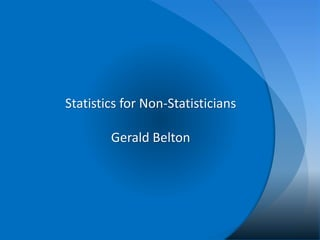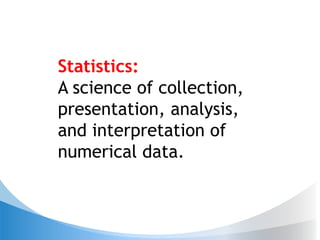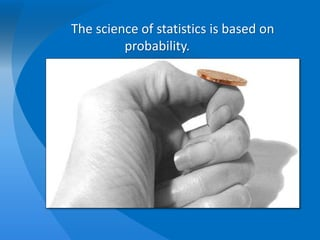Successfully reported this slideshow.

# Statistics for Non-Statisticians

1

Share×
1 of 49
1 of 49

# Statistics for Non-Statisticians

1

Share

A very brief introduction to statistics for non-statisticians, with minimal mathematics. Intended audience is people who work with data and analytics.
Presented at Analytics Forward (https://www.meetup.com/Research-Triangle-Analysts/events/237118943/) on 3/10/18

A very brief introduction to statistics for non-statisticians, with minimal mathematics. Intended audience is people who work with data and analytics.
Presented at Analytics Forward (https://www.meetup.com/Research-Triangle-Analysts/events/237118943/) on 3/10/18

## More Related Content

### Related Books

Free with a 14 day trial from Scribd

See all

### Related Audiobooks

Free with a 14 day trial from Scribd

See all

### Statistics for Non-Statisticians

1. 1. Statistics for Non-Statisticians Gerald Belton
2. 2. Statistics: A science of collection, presentation, analysis, and interpretation of numerical data.
3. 3. The science of statistics is based on probability.
4. 4. Discrete distributions describe data that can only take specific values.
5. 5. A coin toss is an example of a Bernoulli distribution. 0 0.1 0.2 0.3 0.4 0.5 0.6 Heads Tails Probability Bernoulli Distribution
6. 6. A Binomial Distribution results from multiple coin tosses. 0.001 0.0098 0.0439 0.1172 0.2051 0.2461 0.2051 0.1172 0.0439 0.0098 0.001 0 0.05 0.1 0.15 0.2 0.25 0 1 2 3 4 5 6 7 8 9 10 Probability Number of heads Tossing a coin 10 times
7. 7. Rolling one die can be described with a Uniform distribution. 0.167 0.167 0.167 0.167 0.167 0.167 0.000 0.050 0.100 0.150 0.200 0.250 1 2 3 4 5 6 Probability Number Rolled Rolling one die 0.028 0.056 0.083 0.111 0.139 0.167 0.139 0.111 0.083 0.056 0.028 0.000 0.050 0.100 0.150 0.200 0.250 2 3 4 5 6 7 8 9 10 11 12 Probability Number Rolled Rolling two dice
8. 8. Continuous distributions describe data that can take infinitely many values.
9. 9. Rainfall amounts follow an exponential distribution.
10. 10. The Normal Distribution is a very special continuous distribution. 1 2𝜋𝜎2 𝑒 − (𝑥−𝜇)2 2𝜎2
11. 11. Lots of real-world measures are “sort of” normally distributed.
12. 12. Here’s an idealized normal distribution.
13. 13. Here’s an idealized normal distribution.
14. 14. 68% Here’s an idealized normal distribution. σ
15. 15. Here’s an idealized normal distribution. 95% 99.7%
16. 16. Central Limit Theorem makes other distributions “act normal.”
17. 17. Descriptive statistics tell us about the world.
18. 18. Visualizations quickly convey information.
19. 19. Census Map of NC
20. 20. Florence Nightingale
21. 21. Florence Nightingale
22. 22. Minard’s Map
23. 23. Numerical descriptions provide more detail.
24. 24. Location: Mean, Median, Mode
25. 25. Spread: Variance, Std Dev
26. 26. Five number summary > summary(GaltonFathers\$father) Min. 1st Qu. Median Mean 3rd Qu. Max. 62.00 68.00 69.50 69.32 71.00 78.50 >
27. 27. We have tools for looking at the relationship between variables.
28. 28. Correlation
29. 29. Not Causation!
30. 30. Spurious Correlation Example
31. 31. Statistical Inference uses properties of a sample to explain a population. Population Sample StatisticsParameters Sampling Technique Inference
32. 32. Sampling is extremely important.
33. 33. Online Survey Example
34. 34. Simple Random Sample vs. Stratified Random Sample
35. 35. Sample Size vs Precision
36. 36. We use data to build models of reality.
37. 37. Confidence Intervals, Hypothesis Testing, p- value • Null Hypothesis: What we are hoping to disprove. • Alternative Hypothesis: What we hope to prove. • P-value: The probability of observing results at least as extreme as these, if the null hypothesis is true.
38. 38. When we get it wrong α β
39. 39. Another way to remember it
40. 40. Significance is important, but significant results might not be.
41. 41. Significant <> Important
42. 42. P-hacking: false significance Goodheart’s Law: when a measure become a target, it is no longer a measure
43. 43. Measuring weirdness
44. 44. Measuring Weirdness
45. 45. Measuring Weirdness in two dimensions
46. 46. Probability Descriptive Statistics Inference Questions?

### Editor's Notes

• Before we can talk about statistics, we need to talk about probability. Specifically, we need to talk about the two kinds of probability distributions:
• Rolling dice always gives an integer value. A coin toss is either heads or tails. A chart of all the possible outcomes illustrates a discrete distribution.
• Every time you toss a coin, it comes up either heads or tails – unless a seagull snatches it out of the air, or it sticks sideways in the ground. If it’s a fair coin, we expect to get heads 50% of the time and tails 50% of the time. Whenever we have two possible outcomes, we have a Bernoulli Distribution.
• If we toss a coin 10 times and count the number of times it comes up heads, and then do that same thing over and over again, this is how the results should look. We have a very small (but not zero!) chance of 10 heads or 10 tails; five of each is the most likely outcome, and we should see that about 25% of the time.
• We see the same thing with dice. If we roll one die, there are six possible outcomes, and each outcome has a probability of 1/6.
But if we roll two dice, we have 10 possible outcomes; 7 is the most likely outcome, while 2 and 12 are the least likely.
• If we are measuring blood sugar, or temperature, or many other things, the result is a continuous distribution. The number of possible outcomes is infinite (even though it may be bounded).

• This graph shows the number of days in 2017 with various amounts of rainfall at RDU airport. Days with no rain are not included here; We see most rainy days have less than a quarter-inch of rainfall, and as the amount of rain increases, the number of days decreases.
• This curve has some interesting properties… for one thing, its length is infinite but the area under the curve is exactly 1.
• This graph represents the height, in inches, of 205 men measured in England in the1880’s. The data was collected by Francis Galton, a cousin of Charles Darwin. Galton studied the heights of 205 men, their wives, and their adult children.

Galton, F. (1886). Regression Towards Mediocrity in Hereditary Stature Journal of the Anthropological Institute, 15, 246-263
• Here, I’ve colored sections of the curve to show the inflection points. The red part of the curve is concave-down; the blue parts are concave-up. The boundary between red and blue is the inflection point, where the curve changes direction.
• I’ve added a line at the center of the graph, labeled “mu” because statisticians love Greek letters. Mu refers to the population mean, or average, and it coincides with the peak of the normal curve.

I’ve also added vertical lines at the inflection points. The distance from mu to these lines is called the standard deviation, or sigma (because statisticians love Greek letters).

In any normal distribution, 68% of the population will fall within 1 standard deviation of the mean.
• I’ve added lines here for 2 and 3 standard deviations from the mean. 95% of the population falls within 2 (actually, 1.96) standard deviations, and 99.7% within 3 standard deviations.
• How can the sample mean have a distribution? Isn’t it just one number?

If we take REPEATED samples, we will get a different mean from each different sample. So the mean from our random sample is one observation chosen at random from this distribution.
• Enough about probability. Let’s talk about statistics. First, we’ll talk about descriptive statistics. By “descriptive statistics,” we mean numbers that tell us something about a population or a sample.
• I really like data visualizations, they can provide a lot of information in a compact, easily digested form.
• For example, here’s some information about North Carolina from the census bureau. (point out features of this infographic)
• Does anyone know who this is?

Are there any nurses here?
• Yes, that’s Florence Nightingale. Most people know her as the founder of modern nursing, but she was also a pioneer in the use of statistics.
• This is Nightingale’s famous “Diagram of the causes of mortality in the army of the East,” which she made during the Crimean war. The pink areas represent the number of soldier’s who died from battle wounds, the blue areas represent the number of soldiers who died from poor sanitation or infections, and the black areas represent deaths from all other causes. After she insisted that nurses and doctors wash their hands, and after the sanitation commission flushed out the sewers and improved ventilation in the hospitals, the death rate dropped dramatically. This chart was instrumental in bringing about those reforms.
• This is a map drawn by Charles Minard in 1869. Noted information designer Edward Tufte says it "may well be the best statistical graphic ever drawn.”

(point out features of the map)
• Not everything can, or should be, made into a chart. Sometimes you just need to see the numbers. There are certain numbers that are essential to understanding the distribution of a data set.
• Measures of location, or central tendency: mean (arithmetic average), median (half the data are below, half above) and mode (most common occurring number)

Skewed data results from outliers. Consider an extreme example, a village with 100 workers and one factory owner. The workers are each paid \$10/year, and the factory owner makes \$1,000,000 per year. The mean wage is \$9,911 per year, but the median and the mode are both \$10.
• Measures of spread: variance, standard deviation

In the variance formula we measure the distance from each data point to the mean… some will be negative, some positive, so we square them to keep them from cancelling out. Then we add together all of those squared differences, and divide by the number of data points. This average squared distance is called the variance. If we take the square root of the variance, we get the standard deviation.
• Fence: 1-1/2 times the interquartile range from the median. Points beyond the fence are marked as outliers.
• Population examples: Everyone in North Carolina; Adults over 50 with high blood pressure; All of the Medicaid claims filed by a specific provider between 1Jul2017 and 31Dec2017.

Parameters: A number that describes the population: Median age of people in North Carolina; Average Systolic BP of A50+; Amount Medicaid overpayed the provider.

Sample: We can’t measure the entire population, so we draw a random sample.

Statistics: Numbers that describe the sample (rather than the population):

We use statistics measured on a random sample to infer the parameters of the population.
• There are a lot of different sampling methods, but it is important that they be random in order to avoid biasing the results.
• Do we believe the results of this survey? Why?

Website surveys like this are not representative of the population, because the respondents are not chosen at random.
• In a simple random sample, every member of the population has the same probability of being selected.

In a stratified random sample, every member of a subgroup (strata) in the population has the same probability of being selected as every other member of the same subgroup.

• These are formulas for the standard deviation of two different types of data… what they have in common is “n”, the number of observations in the sample. The bigger this number gets, the smaller the spread of the data.
• George Edward Pelham was a British statistician, who has been called "one of the great statistical minds of the 20th century“

All models are wrong: there are no perfect spheres in nature. Some are useful: We can divide the earth’s surface as if it were a sphere, and the results are good enough to locate objects with our GPS systems.
• As we said before, we measure a variable across our sample, calculate a statistic, and use that statistic to estimate the parameter for the population. The result is never exact but the good news is that we can describe just how inexact it is!
• We can describe the inherent uncertainty in our data using confidence intervals; we can use our results to test a hypothesis, specify the results using a p-value.

Definitions: on the slide

Explain graphs briefly
• Type 1 Error: alpha (because we love Greek letters!) is the probability of making a type 1 error. False positive;
Type II Error: beta (because we love Greek letters!) is the probability of making a type 2 error. False negative.
Notice that the null hypothesis is never proven; we either reject it or we fail to reject it. Just like in a courtroom trial, where the defendant is never found innocent, only “not guilty.” Courtroom: Null Hypothesis = Defendant is innocent. Prosecutor has to prove guilt in order to reject the null hypothesis.
• Ha Ha!
• Imagine a clinical experiment where we can conclusively prove that a new drug will lower blood pressure. But it only lowers it by an average of 1 point, say from 140 to 139. The result is statistically significant but nobody cares, because it is not clinically important.
• Clinical trials are set up to look for a “clinically important difference.” Sample size is chosen so that if that difference exists, there will be a specific probability of detecting it. This is called the “power” of the trial.

Power is 1 minus beta. Confidence is 1 minus alpha.
• P: the probability that, if the null hypothesis is true, we would observe results at least as extreme as the ones we have observed.

It is common to use 0.05 as the cutoff for statistical significance, but this is arbitrary.

Also, by increasing the sample size, we can ALWAYS get a result with p < 0.05 or any other arbitrary level.
• Here again is the Galton data on the heights of adult males in England in the 1880’s. It follows a normal distribution (more or less), and we note that there are a couple of men in his sample who are unusually short, and one who is unusually tall. The tall guy here is 6 feet 6 inches, by the way. The mean height is about 69 inches and the standard deviation is 2 and a half inches. Our tall guy is about 3.6 standard deviations taller than the average. Based on our normal distribution we can calculate that he would be taller than 99.98% of the population.
• Just for fun, I’ve added one data point to the graph: Shaq! At 85” tall, Shaq is 6.4 standard deviations above the average. He’s taller than 99.999999% of the population!
• Here we have data points plotted across two correlated variables. The circled point is not an extreme outlier in either dimension, but it’s far away from the mass of spots in the ellipse.

We can generalize this to any number of dimensions, but it’s hard to visualize. But we can express it mathematically, and the difference between the chosen point and the center of the data is called the Mahalanobis distance.